# Chargin with Coulomb l The SI unit of

• Slides: 11Chargin’ with Coulomb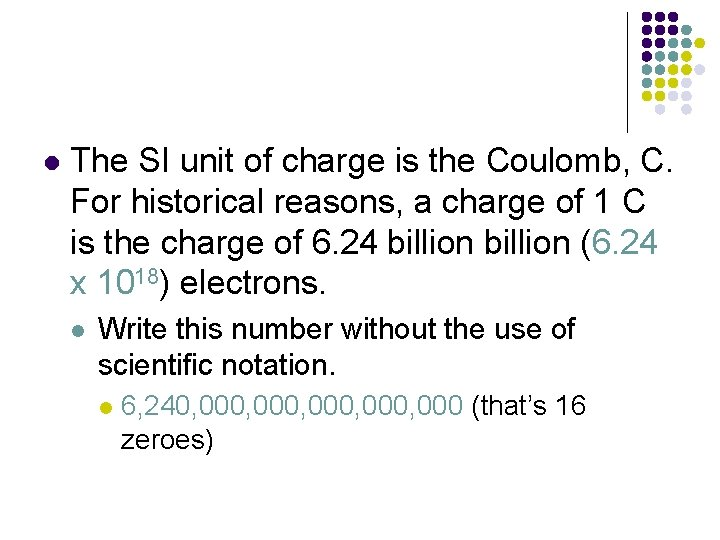l The SI unit of charge is the Coulomb, C. For historical reasons, a charge of 1 C is the charge of 6. 24 billion (6. 24 x 1018) electrons. l Write this number without the use of scientific notation. l 6, 240, 000, 000 (that’s 16 zeroes)l This might seem like a large number of electrons but it represents only the amount of charge which passes through a common 100 W bulb in about one SECOND.l In 1785, CHARLES Coulomb developed Coulomb’s law which relates the amount of electrostatic force between CHARGES.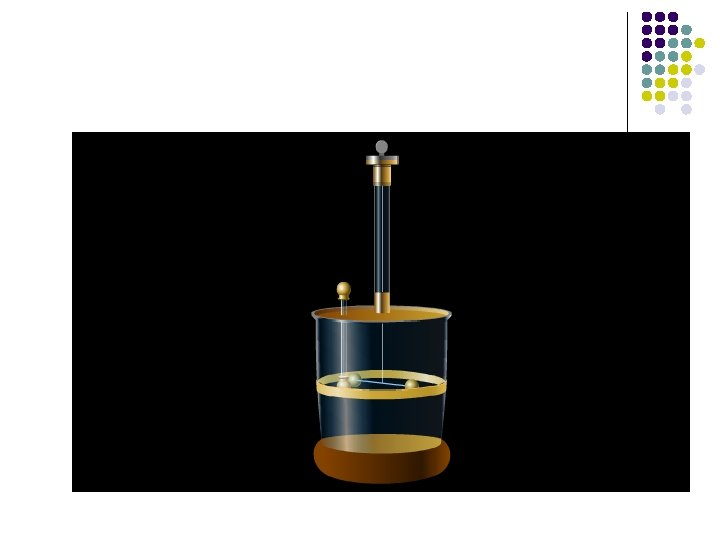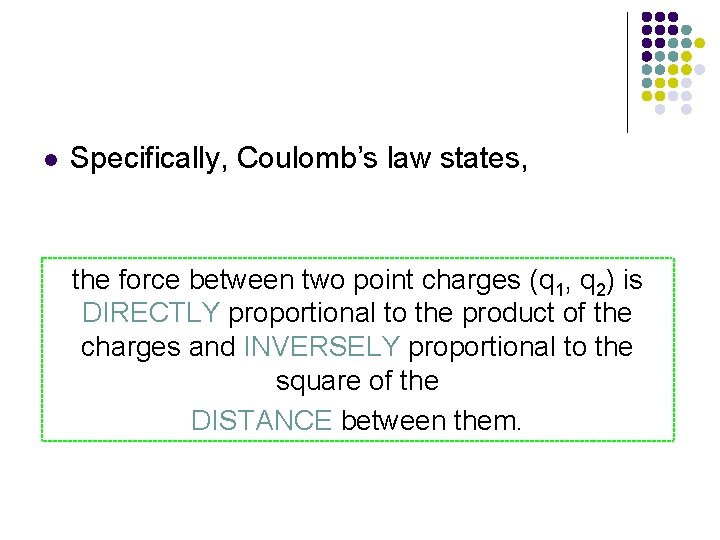l Specifically, Coulomb’s law states, the force between two point charges (q 1, q 2) is DIRECTLY proportional to the product of the charges and INVERSELY proportional to the square of the DISTANCE between them.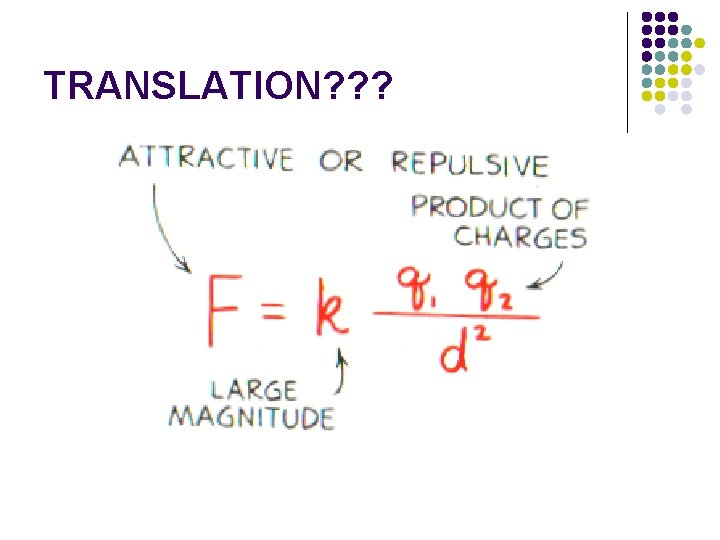TRANSLATION? ? ?l The symbol “k” is a proportionality CONSTANT known as Coulomb’s law CONSTANT. The value of this constant is dependent upon the MAGNITUDE the charged objects are in. l k = 9 x 109 l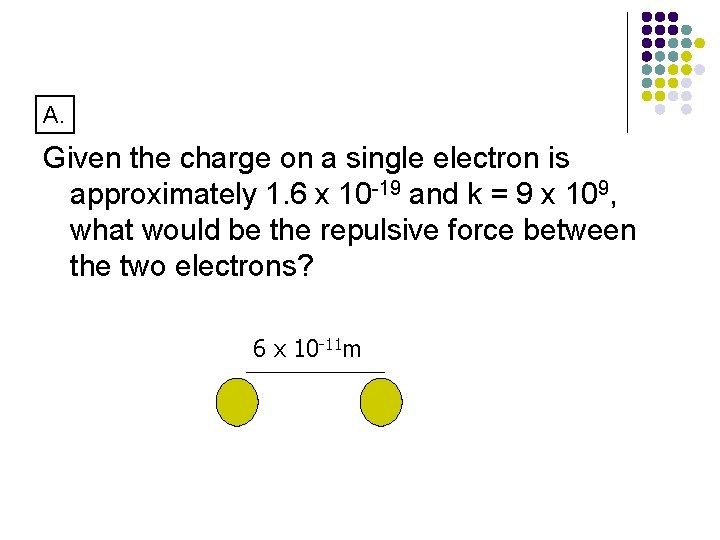A. Given the charge on a single electron is approximately 1. 6 x 10 -19 and k = 9 x 109, what would be the repulsive force between the two electrons? 6 x 10 -11 m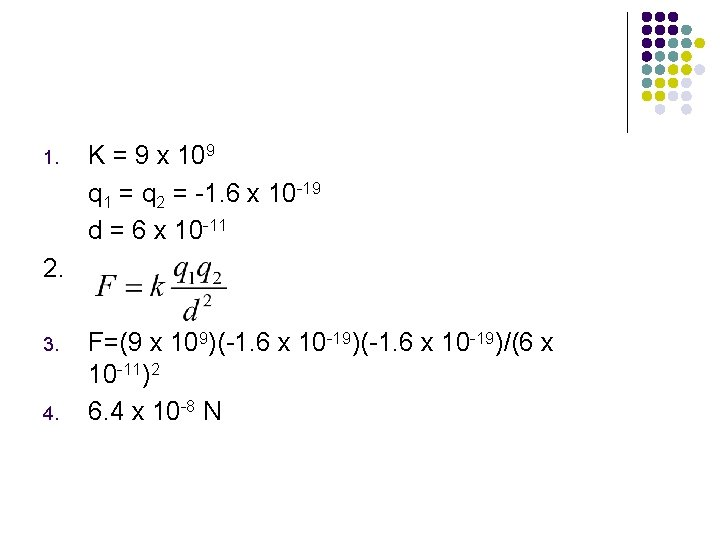1. K = 9 x 109 q 1 = q 2 = -1. 6 x 10 -19 d = 6 x 10 -11 2. 3. 4. F=(9 x 109)(-1. 6 x 10 -19)/(6 x 10 -11)2 6. 4 x 10 -8 Nl HOMEWORK l l l pg 13 worksheet DUE TOMORROW 2/20